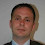## Wednesday, August 25, 2010

### Exploiting the Farkas certificate in Dantzig-Wolfe decomposition

Paul Rubin ask in the comments of his blog post how to exploit a Farkas certificate Dantzig-Wolfe decomposition. I will answer that question here since it is very simple and not well-known. It is also fun to answer.

So let us assume you do

min c_1' x_1 + c_2' x_2
st.  A_1 x_1 + A_2 x_2 = b
B_1 x_1           = f_1              (P)
B_2 x_2           = f_2
x_1, x_2 >= 0

In DW the master problem looks like

min h_1' z_1  + h_2' z_2
st   H_1  z_1 + H_2 z_2 = b          [y]
e' z_1                       = 1          [u_1]
e' z_2    = 1           [u_2]
z_1, z_2 >= 0

e is the vector of all ones. y, u_1 and u_2 are dual variables associated with the constraints.

So it DW you have master problem and I will *NOT* assume that it is feasible. On other hand I will assume
it is infeasible and the LP optimizer provides a Farkas certificate of infeasibility denoted y, u_1 and u_2 i.e.

b'y + u_1 + u_2 > 0,
H_1'y + e u_1 <=0,
H_2'y + e u_2 <= 0

So if you do DW you will have to add columns to the master problem that invalids infeasibility certificate of the master problem.. Finding such column you do using the sub problems

min (0-A_j^T y)' x_j - u_j
st. B_j x_j =f_j
x_j  >= 0

An interpretation of the subproblem is that we find a new column to the master problem that invalidates the Fakas certificate to the master problem as much as possible.

Now it is easy to verify that

• Assume the objective value to a sub problem is negative, then the optimal solution can be used to generate  a new (useful) column to the master problem.
• Now if all the objective values to the problem are nonnegative then the problem (P) is infeasible. Hint: Can you specify a Farkas certificate to (P)?

1.Paul Rubin alerted my to a small typo that has been fixed.

2.There is also a typo in the headline, I think GBD would like to be cited correctly :-)

3.That is correct.

4.I have corrected Dantzigs last name now. Thanks. [If GBD thinks something about his name now I would consider it quite scary :-).]